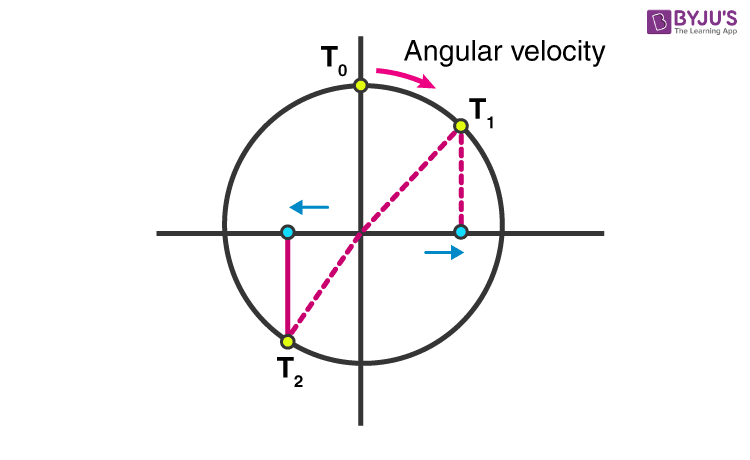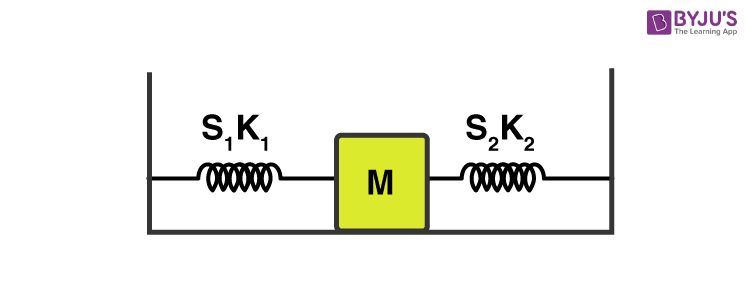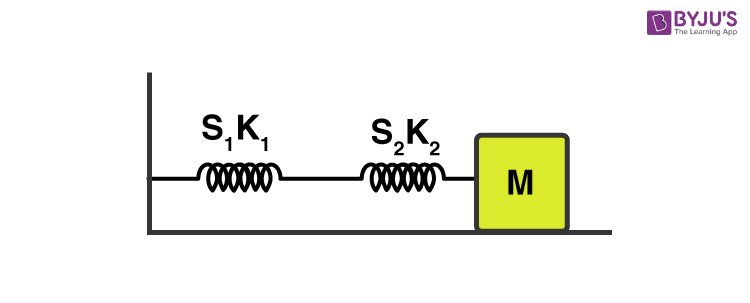Checkout JEE MAINS 2022 Question Paper Analysis : Checkout JEE MAINS 2022 Question Paper Analysis :

# Introduction To Spring Constant

In the last article, we learnt the basic concepts of SHM. Now we shall try to visualise the spring-mass system. Before that, we will see what spring constant is. Force by the action of the spring is given by,

$$\begin{array}{l}F\end{array}$$
=
$$\begin{array}{l}-kx\end{array}$$

k is known as the spring constant or stiffness constant.

Unit of spring constant is N/m.

There are different types of spring. For example torsion spring which works due to turning of the spring.

We can also visualise this spring-mass motion with the help of uniform circular motion.Suppose at

$$\begin{array}{l} t\end{array}$$
=
$$\begin{array}{l} 0 \end{array}$$
the particle is as shown in the figure. If we take the projection of that on the x-axis it is at origin. At
$$\begin{array}{l}t \end{array}$$
=
$$\begin{array}{l} T_1\end{array}$$
, if we take the projection along x-axis it is in positive x-direction and having positive velocity. Similarly, at
$$\begin{array}{l}t\end{array}$$
=
$$\begin{array}{l}T_2\end{array}$$
, the projection is in negative x-axis and having negative velocity. By observing this we can say we take the projection of uniform circular motion on the x-axis it represents an SHM. Sometimes this approach is very useful in solving the numerical problem as compared to equation-based approach.

How to find spring constant when a number of springs are connected together? Suppose we have a situation as shown below:Spring-mass system 1

Suppose the mass is displaced by x towards right, so the force exerted by S2

$$\begin{array}{l}F_2\end{array}$$
=
$$\begin{array}{l}F_2=-k_2x\end{array}$$
(Towards left)

Force exerted by

$$\begin{array}{l}S_1\end{array}$$
,

$$\begin{array}{l}F_1\end{array}$$
=
$$\begin{array}{l}-k_1x\end{array}$$
(Towards left)

So the total restoring force

$$\begin{array}{l}(F)\end{array}$$
=
$$\begin{array}{l} F_1 + F_2\end{array}$$

$$\begin{array}{l}F\end{array}$$
=
$$\begin{array}{l}- (k_1 + k_2) x\end{array}$$

Hence, equivalent

$$\begin{array}{l}k \end{array}$$
=
$$\begin{array}{l}k_1 + k_2\end{array}$$

Using a similar approach try to find equivalent spring constant for the following?Also, the time period for the spring mass system can be shown to be,

$$\begin{array}{l}T\end{array}$$
=
$$\begin{array}{l}2π\sqrt{\frac{m}{k}}\end{array}$$

Where k is the equivalent stiffness constant.

The relationship between the spring constant and the displacement is explained in the video with the help of a spring balance.## Frequently Asked Questions – FAQs

### What is the unit of the spring constant?

The unit of the spring constant is N/m.

### What is a spring?

Spring is an object that can be deformed by force and then return to its original shape when the force is removed.

### What is the formula to find the force by the action of the spring?

The force by the action of the spring is given by the formula: F=-kx

### How is the spring classified based on the displacement due to force applied?

• Constant Force Spring
• Variable Rate Spring
• Linear Spring
• ### What is the formula to find the spring force?

The Spring force formula is given by: F = k(x – x0)

Test your knowledge on Introduction To Spring Constant